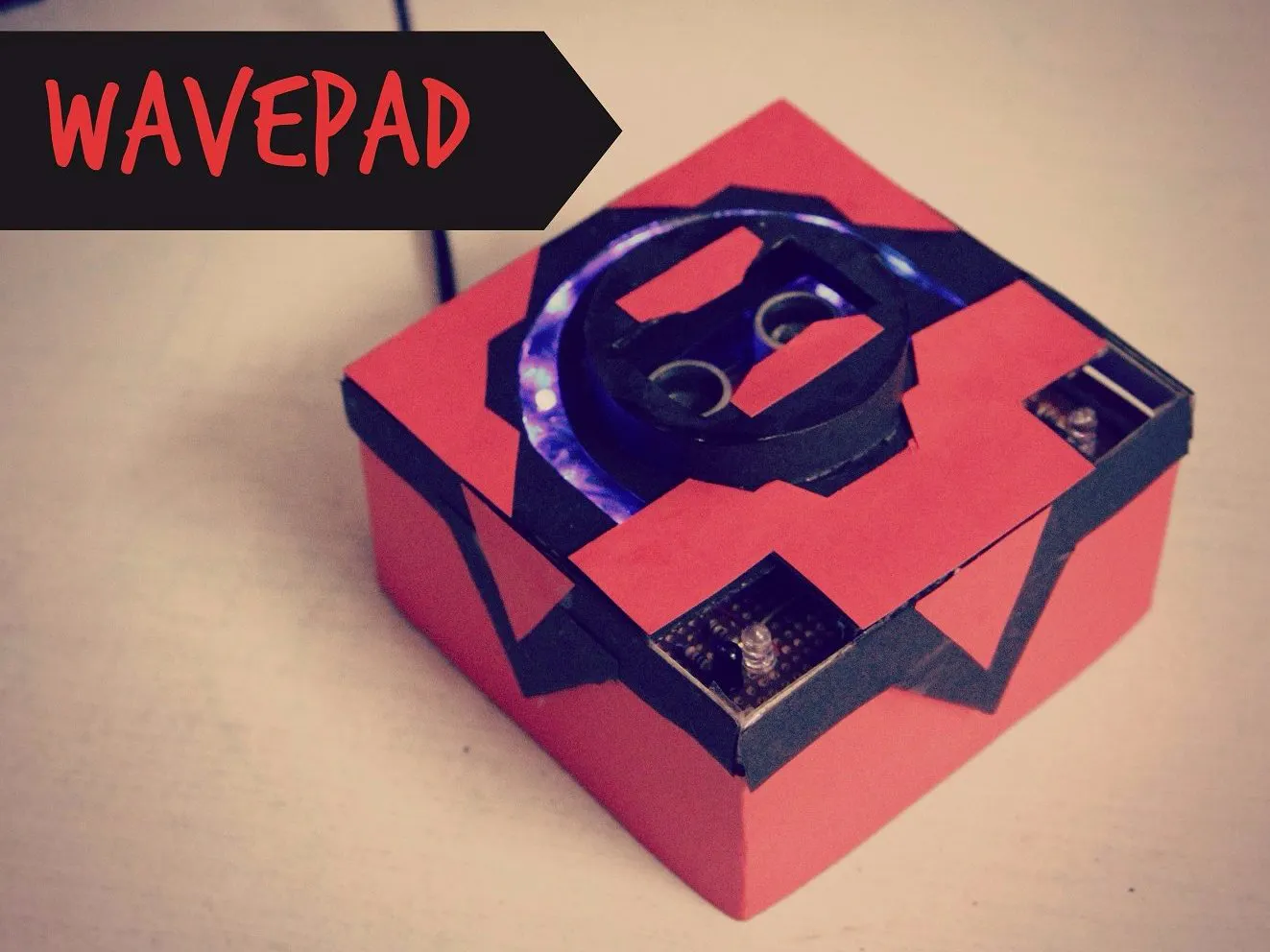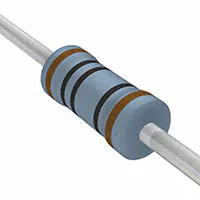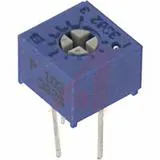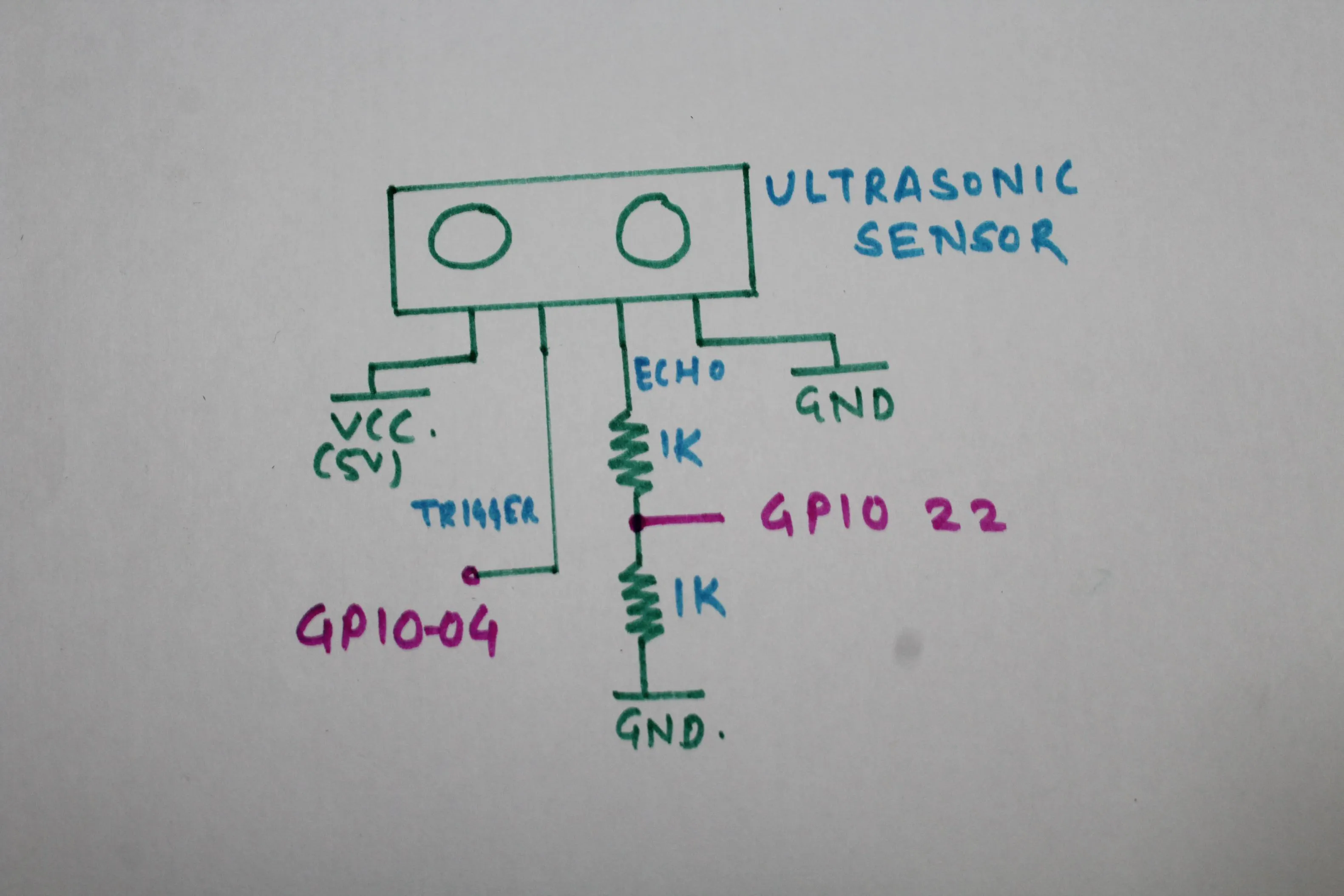Gesture Controlled Raspberry Pi Music Player

IntermediateFull instructions provided12 hours2,990## Things used in this project

### Hardware componentsRaspberry Pi 3 Model B
×1
 Speaker
×1
 Ultrasonic Sensor
×1
 Photodiode
×2
 IR LED
×2Resistor 1k ohm
×18Resistor 10k ohm
×2Resistor 100 ohm
×1Single Turn Potentiometer- 10k ohms
×2
 PCB
×1
 LED Blue
×12
 IC LM324SN
×1Jumper wires (generic)
×30

### Hand tools and fabrication machinesSoldering iron (generic)

## Schematics

### IR sensor pair

It is used to build IR Sensor pair on PCB

### Ultrasonic sensor connection

It is used to connect ultrasonic sensor with voltage divider circuit## Code

Python
python script
```#!/user/bin/python

import os
import glob
import subprocess
import RPi.GPIO as GPIO
import time
from time import sleep

TRIGGER = 4
ECHO = 22
LED1 = 14
LED2 = 15
LED3 = 18
LED4 = 23
LED5 = 24
LED6 = 25
LED7 = 8
LED8 = 7
LED9 = 12
LED10 = 16
LEFT_SENSOR=27
RIGHT_SENSOR=17

GPIO.setmode(GPIO.BCM)
GPIO.setup(LEFT_SENSOR,GPIO.IN)
GPIO.setup(RIGHT_SENSOR,GPIO.IN)

GPIO.setup(TRIGGER,GPIO.OUT)
GPIO.setup(ECHO,GPIO.IN)

GPIO.setup(LED1,GPIO.OUT)
GPIO.setup(LED2,GPIO.OUT)
GPIO.setup(LED3,GPIO.OUT)
GPIO.setup(LED4,GPIO.OUT)
GPIO.setup(LED5,GPIO.OUT)
GPIO.setup(LED6,GPIO.OUT)
GPIO.setup(LED7,GPIO.OUT)
GPIO.setup(LED8,GPIO.OUT)
GPIO.setup(LED9,GPIO.OUT)
GPIO.setup(LED10,GPIO.OUT)

os.chdir('/home/pi/Music')
f = glob.glob('*mp3')
h = len(f)
status = 1
pointer = 0
start = 0
volume = 8
def led():
if volume==1:
GPIO.output(LED1,True)
GPIO.output(LED2,False)
GPIO.output(LED3,False)
GPIO.output(LED4,False)
GPIO.output(LED5,False)
GPIO.output(LED6,False)
GPIO.output(LED7,False)
GPIO.output(LED8,False)
GPIO.output(LED9,False)
GPIO.output(LED10,False)
elif volume==2:
GPIO.output(LED1,True)
GPIO.output(LED2,True)
GPIO.output(LED3,False)
GPIO.output(LED4,False)
GPIO.output(LED5,False)
GPIO.output(LED6,False)
GPIO.output(LED7,False)
GPIO.output(LED8,False)
GPIO.output(LED9,False)
GPIO.output(LED10,False)
elif volume==3:
GPIO.output(LED1,True)
GPIO.output(LED2,True)
GPIO.output(LED3,True)
GPIO.output(LED4,False)
GPIO.output(LED5,False)
GPIO.output(LED6,False)
GPIO.output(LED7,False)
GPIO.output(LED8,False)
GPIO.output(LED9,False)
GPIO.output(LED10,False)
elif volume==4:
GPIO.output(LED1,True)
GPIO.output(LED2,True)
GPIO.output(LED3,True)
GPIO.output(LED4,True)
GPIO.output(LED5,False)
GPIO.output(LED6,False)
GPIO.output(LED7,False)
GPIO.output(LED8,False)
GPIO.output(LED9,False)
GPIO.output(LED10,False)
elif volume==5:
GPIO.output(LED1,True)
GPIO.output(LED2,True)
GPIO.output(LED3,True)
GPIO.output(LED4,True)
GPIO.output(LED5,True)
GPIO.output(LED6,False)
GPIO.output(LED7,False)
GPIO.output(LED8,False)
GPIO.output(LED9,False)
GPIO.output(LED10,False)
elif volume==6:
GPIO.output(LED1,True)
GPIO.output(LED2,True)
GPIO.output(LED3,True)
GPIO.output(LED4,True)
GPIO.output(LED5,True)
GPIO.output(LED6,True)
GPIO.output(LED7,False)
GPIO.output(LED8,False)
GPIO.output(LED9,False)
GPIO.output(LED10,False)
elif volume==7:
GPIO.output(LED1,True)
GPIO.output(LED2,True)
GPIO.output(LED3,True)
GPIO.output(LED4,True)
GPIO.output(LED5,True)
GPIO.output(LED6,True)
GPIO.output(LED7,True)
GPIO.output(LED8,False)
GPIO.output(LED9,False)
GPIO.output(LED10,False)
elif volume==8:
GPIO.output(LED1,True)
GPIO.output(LED2,True)
GPIO.output(LED3,True)
GPIO.output(LED4,True)
GPIO.output(LED5,True)
GPIO.output(LED6,True)
GPIO.output(LED7,True)
GPIO.output(LED8,True)
GPIO.output(LED9,False)
GPIO.output(LED10,False)
elif volume==9:
GPIO.output(LED1,True)
GPIO.output(LED2,True)
GPIO.output(LED3,True)
GPIO.output(LED4,True)
GPIO.output(LED5,True)
GPIO.output(LED6,True)
GPIO.output(LED7,True)
GPIO.output(LED8,True)
GPIO.output(LED9,True)
GPIO.output(LED10,False)
elif volume==10:
GPIO.output(LED1,True)
GPIO.output(LED2,True)
GPIO.output(LED3,True)
GPIO.output(LED4,True)
GPIO.output(LED5,True)
GPIO.output(LED6,True)
GPIO.output(LED7,True)
GPIO.output(LED8,True)
GPIO.output(LED9,True)
GPIO.output(LED10,True)

def distance():
GPIO.output(TRIGGER,True)
time.sleep(0.00001)
GPIO.output(TRIGGER,False)
StartTime = time.time()
StopTime = time.time()
while GPIO.input(ECHO) == 0:
StartTime = time.time()
while GPIO.input(ECHO) == 1:
StopTime = time.time()
TimeElapsed = StopTime-StartTime
distance=(TimeElapsed*34300)/2
return distance

if __name__=='__main__':
try:
for counter in range(1,10):
volume = counter
led()
sleep(0.1)
for counter in range(1,9):
volume = 10-counter
led()
sleep(0.1)
for counter in range(1,8):
volume = counter
led()
sleep(0.1)
while True:

led()
dist = int(distance())
if(status==1):
player = subprocess.Popen(["omxplayer",f[pointer]],stdin=subprocess.PIPE)
fi = player.poll()
status = 0
start = 0
volume = 8
if(dist>=0 and dist<=35):
vol = dist/3
if(vol>volume):
for counter in range(1,vol-volume):
player.stdin.write("+")
volume = volume + 1
led()
sleep(0.1)
elif(vol<volume):
for counter in range(1,volume-vol):
player.stdin.write("-")
volume = volume - 1
led()
sleep(0.1)
if(GPIO.input(LEFT_SENSOR)==True and GPIO.input(RIGHT_SENSOR)==True):
sleep(0.5)
fi = player.poll()
if fi!=0:
player.stdin.write("p")
elif(GPIO.input(LEFT_SENSOR)==True):
for counter in range(0,10000):
if(GPIO.input(RIGHT_SENSOR)==True):
if start==0:
player.stdin.write("q")
status = 1
pointer = pointer +1
if(pointer>h-1):
pointer = 0
break
sleep(0.0001)
elif(GPIO.input(RIGHT_SENSOR)==True):
for counter in range(0,10000):
if(GPIO.input(LEFT_SENSOR)==True):
if(start==0):
player.stdin.write("q")
status = 1
pointer = pointer - 1
if(pointer<0):
pointer = h-1
break
sleep(0.0001)
else:
fi = player.poll()
if(fi==0 and start==0):
status = 1
pointer = pointer +1
if(pointer>h-1):
pointer = 0
sleep(0.1)
except KeyboardInterrupt:
print("Stopped by user")
GPIO.cleanup()

```

## Credits

### Tejas Ozarkar

2 projects • 5 followers
I'm android developer and robotics enthusiast.